Earn 20 XP

## Learning Objectives

• Conditional Statement
• Dictionary

## Conditional Statement: if-elif-else

• In the real world, we commonly evaluate information around us and then choose one course of action or another based on what we observe:
If the weather is nice, then I'll go for a walk. (It's implied that I won't go for a walk if the weather isn't nice.)
• In a Python program, the if statement is how you perform this decision-making. It allows for the conditional execution of a statement or group of statements based on the value of an expression.

### If

• Syntax (how to write If statement in Python?) -
``````1
2
``````if test expression/condition:
statement(s)
``````
• Here, the program evaluates the test expression and will execute statement(s) only if the test expression is True.
• If the test expression is False, the statement(s) is not executed.
• In Python, the body of the if statement is indicated by the indentation. The body starts with an indentation, and the first unindented line marks the end.
• Python interprets non-zero values as True (even negative values). None and 0 are interpreted as False.
• Let's understand this with a few examples: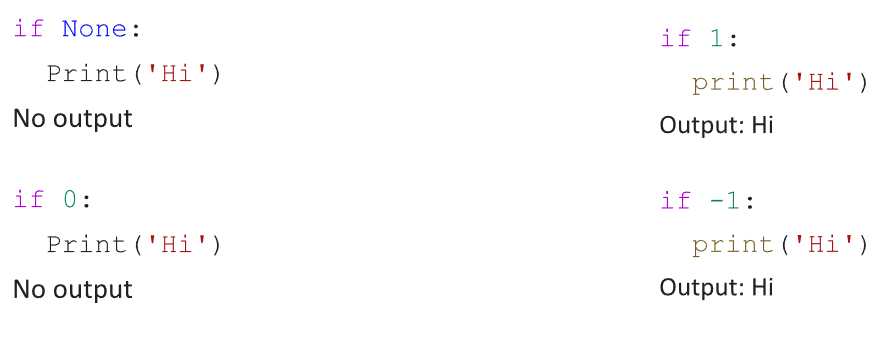• Flowchart: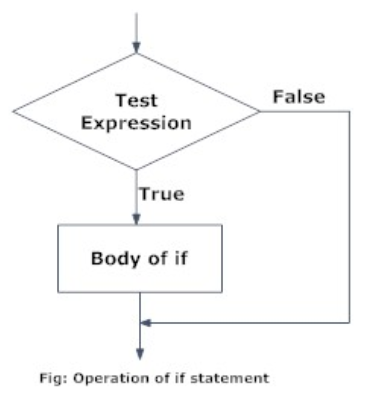• Example: Python program to detect if a number is even. (sign % tells us the remainder of an expression. Any number with a remainder of 0 after dividing by 2 must be even.)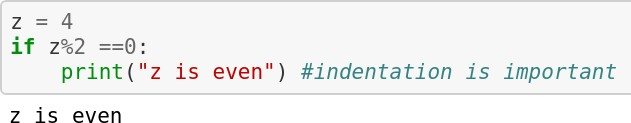### If-else

• Syntax -
``````1
2
3
4
``````if test expression:
Body of if
else:
Body of else
``````
• The if-else statement evaluates test expression and will execute the body of if only when the test condition is True.

• If the condition is False, the body of else is executed. Indentation is used to separate the blocks.

• Flowchart: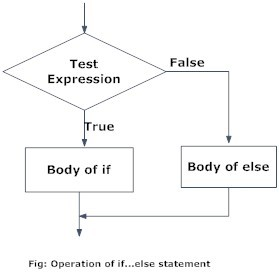• Example: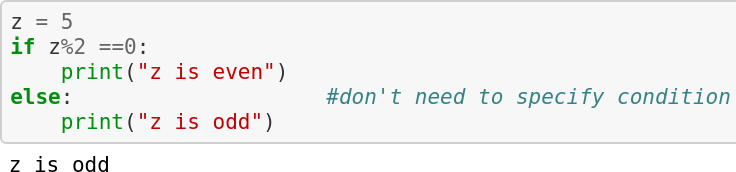### If-elif-else

• Syntax -
``````1
2
3
4
5
6
``````if test expression:
Body of if
elif test expression:
Body of elif
else:
Body of else
``````
• The elif is short for else if. It allows us to check for multiple expressions.

• If the condition for if is False, it checks the condition of the next elif block and so on.

• If all the conditions are False, the body of else is executed.

• Only one block among the several if-elif-else blocks is executed according to the condition.

• The if block can have only one else block. But it can have multiple elif blocks.

• Flowchart: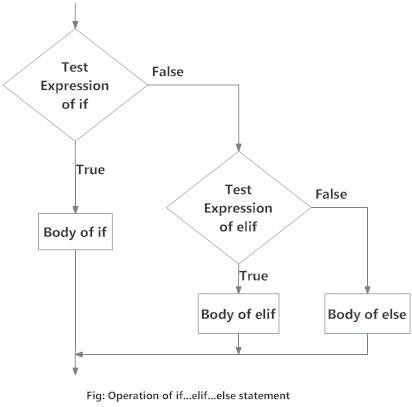• Example: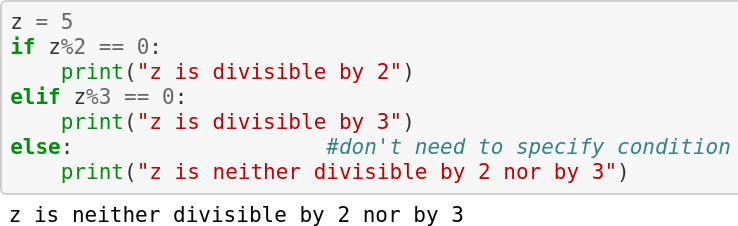• Now, let's try to understand the above example.

• We first assigned the value 5 to x
• The control shifts to the following line where x%2 is checked. 5 is not divisible by 2, so the control doesn't shift to the body of if.
• Then, the elif statement: x%3 is executed. Since 5 is not divisible by 3, the body of elif is not executed.
• Finally, the else statement is executed, and the control shifts to the body of the else statement. The print statement ("z is neither divisible by 2 nor by 3" is executed.
• Point to be noted: The conditions are checked in a top to bottom order. If any of the above if or elif condition is True, it'll be executed, and no other conditions will be checked.

• Can you figure out what z=6 will print in the given example?

## Dictionary

• Dictionary is an unordered collection of key-value pairs.
• Real word dictionaries are a good analogy to understand them: they contain a list of items(words), and each item has a key(the word) and a value(the word's meaning).
• It generally is used when we have a massive amount of data.
• It is defined within braces, with each item in the form of key: value pairs. Syntax –
``````1
2
3
4
``````my_dict = {
key1:value1,
key2:value2,
}
``````

### Dictionary

• The keys in a dictionary must always be unique and immutable. This is the reason dictionary keys can be String but not List.
• On the other hand, Values in a dictionary can be of any datatype and can be duplicated
• Dictionary keys are case sensitive; same name but different cases of Key will be treated distinctly.
• Example: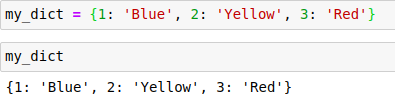### Looping over Dictionary

• Let's say we have a dictionary containing countries as keys and their populations as values.

• For looping through a dictionary, we use a method called items( ). Like enumerate, it gives us both the keys and values of a dictionary.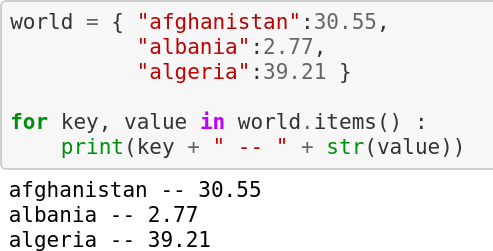For additional practice on dictionaries, visit: https://www.w3schools.com/python/python\_dictionaries.asp

### Let's Practice

1. Take values of length and breadth of a rectangle from the user and check if it is a square.
2. Take two int values from the user and print the greatest among them.
3. Write a program to read a candidate's age and determine whether they are eligible to cast their vote.
4. Write a Python program to add a key to a dictionary.
Sample Dictionary : {0: 10, 1: 20}
Expected Result : {0: 10, 1: 20, 2: 30}
5. Below are two lists; convert them into a dictionary. keys = ['Ten', 'Twenty', 'Thirty']
values = [10, 20, 30]
Expected output:
{'Ten': 10, 'Twenty': 20, 'Thirty': 30}
6. Access the value of key 'history'
``````1
2
3
4
5
6
7
8
9
10
11
``````sampleDict = {
"class":{
"student":{
"name":"Mike",
"marks":{
"physics":70,
"history":80
}
}
}
}
``````

Expected output:
80

1. Given the following dictionary:
inventory = {
'gold' : 500,
'pouch' : ['flint', 'twine', 'gemstone'],